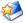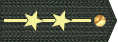# [原创]长征五号火箭研制的瓶颈问题本质就是YF77氢氧发动机的可靠性问题

2018 11 28

打赏
收藏文本
0
0
2018/11/29 9:06:13

## 网友回复

左箭头-小图标

[size=10.5]第四节，研制长征五号火箭侥幸心态要不得。[size=10.5]
[size=10.5]
[size=10.5]长征五号火箭的研制充满着太多自相矛盾的地方，一方面想学习欧洲阿里安五与原苏联能源火箭的技术路径要搞“一级半”构型入轨的高大上方案。而另一方面却又不舍得投入巨资进行[size=10.5]YF77[size=10.5]氢氧发动机的高强度地面试车。[size=10.5]
[size=10.5]
[size=10.5]全球走一级半构型入轨的火箭，其所使用的主芯级氢氧发动机，从[size=10.5]SSME[size=10.5]、[size=10.5]RD0120[size=10.5]到火神[size=10.5]1[size=10.5]，到火箭首飞之前那一个的地面试车累积时间没有超过[size=10.5]10[size=10.5]万秒的巨大程度？！而中国的长征五号火箭在首飞之前的[size=10.5]2014[size=10.5]年，地面试车累积时间也还只有[size=10.5]3[size=10.5]万秒左右。结果长征五号遥[size=10.5]1[size=10.5]火箭发射侥幸成功，而遥[size=10.5]2[size=10.5]火箭发射就直接失败了，原因就是出在[size=10.5]YF77[size=10.5]氢氧发动机的故障身上。[size=10.5]
[size=10.5]
[size=10.5]因此长征五号火箭的研制明显是“步子大了扯到蛋”的例子。其的整个项目从立项、管理到运营风险控制都大量充斥着投机取巧与侥幸蒙滚过关的“病态投机心理”。结果硬生生将长征五号火箭的研制搞成了一锅“夹生饭”，让国家骑虎难下。这一点必须给予严厉批评，以警示后人。[size=10.5]
[size=10.5]
[size=10.5]为今之计，就只有两条路可走了。[size=10.5]
[size=10.5]
[size=10.5]一，就是不惜代价、不惜成本、不惜时间全力推进[size=10.5]YF77[size=10.5]氢氧发动机的[size=10.5]10[size=10.5]万秒地面试车累积工程，并将[size=10.5]1[size=10.5]次性长程地面试车考核通过时间坚决提升到[size=10.5]1500[size=10.5]秒的严格程度（即与[size=10.5]YF100[size=10.5]煤油发动机的[size=10.5]3[size=10.5]倍工作时间长程试车考核相同的水平）。[size=10.5]
[size=10.5]
[size=10.5]二，就是改走日本[size=10.5]H2A[size=10.5]、[size=10.5]H2B[size=10.5]火箭的技术发展路径。即将[size=10.5]YF77[size=10.5]氢氧发动机主芯级的持续工作时间缩小为[size=10.5]350[size=10.5]秒左右。再走“二级半”构型方案来执行[size=10.5]LEO[size=10.5]、[size=10.5]SSO[size=10.5]与[size=10.5]GTO[size=10.5]的发射任务。[size=10.5]
[size=10.5]
[size=10.5]除此两种方案之外，再别无他途。[size=10.5]
[size=10.5]
[size=10.5]陈天（高凉陈君）[size=10.5]
[size=10.5]
[size=10.5]2018[size=10.5]年[size=10.5]11[size=10.5]月[size=10.5]28[size=10.5]日[size=10.5]
[size=10.5]

2018/11/29 11:58:02
•• 军号：3376814
• 工分:10822
左箭头-小图标

为什么拍长时间试车呢？

难道会台毁机亡？

2018/11/29 10:06:39
•• 军号：3376814
• 工分:10822
左箭头-小图标

这点支持。

学习RD0120那种不下试车台的无限时的精神。

2018/11/29 9:39:33

总页数11页 [共有4条记录] 分页：

1
对[原创]长征五号火箭研制的瓶颈问题本质就是YF77氢氧发动机的可靠性问题回复